# Arrays

An array is a series of elements of the same type placed in contiguous memory locations that can be individually referenced by adding an index to a unique identifier.

That means that, for example, five values of type `int` can be declared as an array without having to declare 5 different variables (each with its own identifier). Instead, using an array, the five `int` values are stored in contiguous memory locations, and all five can be accessed using the same identifier, with the proper index.

For example, an array containing 5 integer values of type `int` called `foo` could be represented as: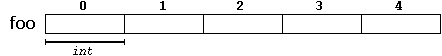where each blank panel represents an element of the array. In this case, these are values of type `int`. These elements are numbered from 0 to 4, being 0 the first and 4 the last; In C++, the first element in an array is always numbered with a zero (not a one), no matter its length.

Like a regular variable, an array must be declared before it is used. A typical declaration for an array in C++ is:

``` type name [elements]; ```
where `type` is a valid type (such as `int`, `float`...), `name` is a valid identifier and the `elements` field (which is always enclosed in square brackets `[]`), specifies the length of the array in terms of the number of elements.

Therefore, the `foo` array, with five elements of type `int`, can be declared as:

 `` `` ``int foo ;``

NOTE: The `elements` field within square brackets `[]`, representing the number of elements in the array, must be a constant expression, since arrays are blocks of static memory whose size must be determined at compile time, before the program runs.

### Initializing arrays

By default, regular arrays of local scope (for example, those declared within a function) are left uninitialized. This means that none of its elements are set to any particular value; their contents are undetermined at the point the array is declared.

But the elements in an array can be explicitly initialized to specific values when it is declared, by enclosing those initial values in braces {}. For example:

 `` `` ``int foo  = { 16, 2, 77, 40, 12071 }; ``

This statement declares an array that can be represented like this:The number of values between braces `{}` shall not be greater than the number of elements in the array. For example, in the example above, `foo` was declared having 5 elements (as specified by the number enclosed in square brackets, `[]`), and the braces `{}` contained exactly 5 values, one for each element. If declared with less, the remaining elements are set to their default values (which for fundamental types, means they are filled with zeroes). For example:

 `` `` ``int bar  = { 10, 20, 30 }; ``

Will create an array like this:The initializer can even have no values, just the braces:

 `` `` ``int baz  = { }; ``

This creates an array of five `int` values, each initialized with a value of zero: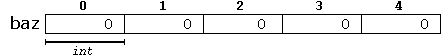When an initialization of values is provided for an array, C++ allows the possibility of leaving the square brackets empty `[]`. In this case, the compiler will assume automatically a size for the array that matches the number of values included between the braces `{}`:

 `` `` ``int foo [] = { 16, 2, 77, 40, 12071 };``

After this declaration, array `foo` would be 5 `int` long, since we have provided 5 initialization values.

Finally, the evolution of C++ has led to the adoption of universal initialization also for arrays. Therefore, there is no longer need for the equal sign between the declaration and the initializer. Both these statements are equivalent:

 ``12`` ``````int foo[] = { 10, 20, 30 }; int foo[] { 10, 20, 30 }; ``````

Static arrays, and those declared directly in a namespace (outside any function), are always initialized. If no explicit initializer is specified, all the elements are default-initialized (with zeroes, for fundamental types).

### Accessing the values of an array

The values of any of the elements in an array can be accessed just like the value of a regular variable of the same type. The syntax is:

`name[index]`
Following the previous examples in which `foo` had 5 elements and each of those elements was of type `int`, the name which can be used to refer to each element is the following:For example, the following statement stores the value 75 in the third element of `foo`:

 `` `` ``foo  = 75;``

and, for example, the following copies the value of the third element of `foo` to a variable called `x`:

 `` `` ``x = foo;``

Therefore, the expression `foo` is itself a variable of type `int`.

Notice that the third element of `foo` is specified `foo`, since the first one is `foo`, the second one is `foo`, and therefore, the third one is `foo`. By this same reason, its last element is `foo`. Therefore, if we write `foo`, we would be accessing the sixth element of `foo`, and therefore actually exceeding the size of the array.

In C++, it is syntactically correct to exceed the valid range of indices for an array. This can create problems, since accessing out-of-range elements do not cause errors on compilation, but can cause errors on runtime. The reason for this being allowed will be seen in a later chapter when pointers are introduced.

At this point, it is important to be able to clearly distinguish between the two uses that brackets `[]` have related to arrays. They perform two different tasks: one is to specify the size of arrays when they are declared; and the second one is to specify indices for concrete array elements when they are accessed. Do not confuse these two possible uses of brackets `[]` with arrays.

 ``12`` ``````int foo; // declaration of a new array foo = 75; // access to an element of the array. ``````

The main difference is that the declaration is preceded by the type of the elements, while the access is not.

Some other valid operations with arrays:

 ``1234`` ``````foo = a; foo[a] = 75; b = foo [a+2]; foo[foo[a]] = foo + 5;``````

For example:

 ``12345678910111213141516`` ``````// arrays example #include using namespace std; int foo [] = {16, 2, 77, 40, 12071}; int n, result=0; int main () { for ( n=0 ; n<5 ; ++n ) { result += foo[n]; } cout << result; return 0; }`````` `12206`

### Multidimensional arrays

Multidimensional arrays can be described as "arrays of arrays". For example, a bidimensional array can be imagined as a two-dimensional table made of elements, all of them of a same uniform data type.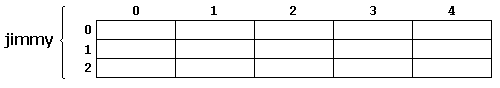`jimmy` represents a bidimensional array of 3 per 5 elements of type `int`. The C++ syntax for this is:

 `` `` ``int jimmy ;``

and, for example, the way to reference the second element vertically and fourth horizontally in an expression would be:

 `` `` ``jimmy``(remember that array indices always begin with zero).

Multidimensional arrays are not limited to two indices (i.e., two dimensions). They can contain as many indices as needed. Although be careful: the amount of memory needed for an array increases exponentially with each dimension. For example:

 `` `` ``char century ;``

declares an array with an element of type `char` for each second in a century. This amounts to more than 3 billion `char`! So this declaration would consume more than 3 gigabytes of memory!

At the end, multidimensional arrays are just an abstraction for programmers, since the same results can be achieved with a simple array, by multiplying its indices:

 ``12`` ``````int jimmy ; // is equivalent to int jimmy ; // (3 * 5 = 15) ``````

With the only difference that with multidimensional arrays, the compiler automatically remembers the depth of each imaginary dimension. The following two pieces of code produce the exact same result, but one uses a bidimensional array while the other uses a simple array:

multidimensional arraypseudo-multidimensional array
```#define WIDTH 5 #define HEIGHT 3 int jimmy [HEIGHT][WIDTH]; int n,m; int main () { for (n=0; n<HEIGHT; n++) for (m=0; m<WIDTH; m++) { jimmy[n][m]=(n+1)*(m+1); } }``` ```#define WIDTH 5 #define HEIGHT 3 int jimmy [HEIGHT * WIDTH]; int n,m; int main () { for (n=0; n<HEIGHT; n++) for (m=0; m<WIDTH; m++) { jimmy[n*WIDTH+m]=(n+1)*(m+1); } }```

None of the two code snippets above produce any output on the screen, but both assign values to the memory block called jimmy in the following way: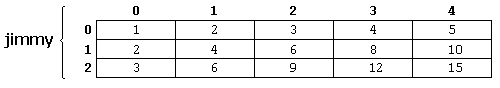Note that the code uses defined constants for the width and height, instead of using directly their numerical values. This gives the code a better readability, and allows changes in the code to be made easily in one place.

### Arrays as parameters

At some point, we may need to pass an array to a function as a parameter. In C++, it is not possible to pass the entire block of memory represented by an array to a function directly as an argument. But what can be passed instead is its address. In practice, this has almost the same effect, and it is a much faster and more efficient operation.

To accept an array as parameter for a function, the parameters can be declared as the array type, but with empty brackets, omitting the actual size of the array. For example:

 `` `` ``void procedure (int arg[])``

This function accepts a parameter of type "array of `int`" called `arg`. In order to pass to this function an array declared as:

 `` `` ``int myarray ;``

it would be enough to write a call like this:

 `` `` ``procedure (myarray);``

Here you have a complete example:

 ``1234567891011121314151617`` ``````// arrays as parameters #include using namespace std; void printarray (int arg[], int length) { for (int n=0; n

In the code above, the first parameter (`int arg[]`) accepts any array whose elements are of type `int`, whatever its length. For that reason, we have included a second parameter that tells the function the length of each array that we pass to it as its first parameter. This allows the for loop that prints out the array to know the range to iterate in the array passed, without going out of range.

In a function declaration, it is also possible to include multidimensional arrays. The format for a tridimensional array parameter is:

 `` `` ``base_type[][depth][depth]``

For example, a function with a multidimensional array as argument could be:

 `` `` ``void procedure (int myarray[])``

Notice that the first brackets `[]` are left empty, while the following ones specify sizes for their respective dimensions. This is necessary in order for the compiler to be able to determine the depth of each additional dimension.

In a way, passing an array as argument always loses a dimension. The reason behind is that, for historical reasons, arrays cannot be directly copied, and thus what is really passed is a pointer. This is a common source of errors for novice programmers. Although a clear understanding of pointers, explained in a coming chapter, helps a lot.

### Library arrays

The arrays explained above are directly implemented as a language feature, inherited from the C language. They are a great feature, but by restricting its copy and easily decay into pointers, they probably suffer from an excess of optimization.

To overcome some of these issues with language built-in arrays, C++ provides an alternative array type as a standard container. It is a type template (a class template, in fact) defined in header `<array>`.

Containers are a library feature that falls out of the scope of this tutorial, and thus the class will not be explained in detail here. Suffice it to say that they operate in a similar way to built-in arrays, except that they allow being copied (an actually expensive operation that copies the entire block of memory, and thus to use with care) and decay into pointers only when explicitly told to do so (by means of its member `data`).

Just as an example, these are two versions of the same example using the language built-in array described in this chapter, and the container in the library:

language built-in arraycontainer library array
```#include <iostream> using namespace std; int main() { int myarray = {10,20,30}; for (int i=0; i<3; ++i) ++myarray[i]; for (int elem : myarray) cout << elem << '\n'; }``` ```#include <iostream> #include <array> using namespace std; int main() { array<int,3> myarray {10,20,30}; for (int i=0; i<myarray.size(); ++i) ++myarray[i]; for (int elem : myarray) cout << elem << '\n'; } ```

As you can see, both kinds of arrays use the same syntax to access its elements: `myarray[i]`. Other than that, the main differences lay on the declaration of the array, and the inclusion of an additional header for the library array. Notice also how it is easy to access the size of the library array.Previous:Name visibility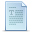IndexNext:Character sequences